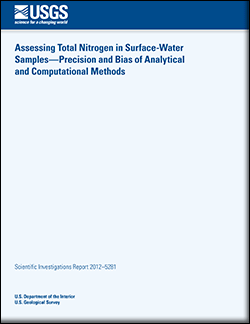Scientific Investigations Report 2012–5281

## Assessing Total Nitrogen in Surface-Water Samples—Precision and Bias of Analytical and Computational Methods

###Abstract

The characterization of total-nitrogen (TN) concentrations is an important component of many surface-water-quality programs. However, three widely used methods for the determination of total nitrogen—(1) derived from the alkaline-persulfate digestion of whole-water samples (TN-A); (2) calculated as the sum of total Kjeldahl nitrogen and dissolved nitrate plus nitrite (TN-K); and (3) calculated as the sum of dissolved nitrogen and particulate nitrogen (TN-C)—all include inherent limitations. A digestion process is intended to convert multiple species of nitrogen that are present in the sample into one measureable species, but this process may introduce bias. TN-A results can be negatively biased in the presence of suspended sediment, and TN-K data can be positively biased in the presence of elevated nitrate because some nitrate is reduced to ammonia and is therefore counted twice in the computation of total nitrogen. Furthermore, TN-C may not be subject to bias but is comparatively imprecise. In this study, the effects of suspended-sediment and nitrate concentrations on the performance of these TN methods were assessed using synthetic samples developed in a laboratory as well as a series of stream samples. A 2007 laboratory experiment measured TN-A and TN-K in nutrient-fortified solutions that had been mixed with varying amounts of sediment-reference materials. This experiment identified a connection between suspended sediment and negative bias in TN-A and detected positive bias in TN-K in the presence of elevated nitrate. A 2009–10 synoptic-field study used samples from 77 stream-sampling sites to confirm that these biases were present in the field samples and evaluated the precision and bias of TN methods.

The precision of TN-C and TN-K depended on the precision and relative amounts of the TN-component species used in their respective TN computations. Particulate nitrogen had an average variability (as determined by the relative standard deviation) of 13 percent. However, because particulate nitrogen constituted only 14 percent, on average, of TN-C, the precision of the TN-C method approached that of the method for dissolved nitrogen (2.3 percent). On the other hand, total Kjeldahl nitrogen (having a variability of 7.6 percent) constituted an average of 40 percent of TN-K, suggesting that the reduced precision of the Kjeldahl digestion may affect precision of the TN-K estimates. For most samples, the precision of TN computed as TN-C would be better (lower variability) than the precision of TN-K. In general, TN-A precision (having a variability of 2.1 percent) was superior to TN-C and TN-K methods.

The laboratory experiment indicated that negative bias in TN-A was present across the entire range of sediment concentration and increased as sediment concentration increased. This suggested that reagent limitation was not the predominant cause of observed bias in TN-A. Furthermore, analyses of particulate nitrogen present in digest residues provided an almost complete accounting for the nitrogen that was underestimated by alkaline-persulfate digestion. This experiment established that, for the reference materials at least, negative bias in TN-A was caused primarily by the sequestration of some particulate nitrogen that was refractory to the digestion process. TN-K biases varied between positive and negative values in the laboratory experiment. Positive bias in TN-K is likely the result of the unintended reduction of a small and variable amount of nitrate to ammonia during the Kjeldahl digestion process. Negative TN-K bias may be the result of the sequestration of a portion of particulate nitrogen during the digestion process.

Negative bias in TN-A was present across the entire range of suspended-sediment concentration (1 to 14,700 milligrams per liter [mg/L]) in the synoptic-field study, with relative bias being nearly as great at sediment concentrations below 10 mg/L (median of -3.5 percent) as that observed at sediment concentrations up to 750 mg/L (median of -4.4 percent). This lent support to the laboratory-experiment finding that some particulate nitrogen is sequestered during the digestion process, and demonstrated that negative TN-A bias was present in samples with very low suspended-sediment concentrations. At sediment concentrations above 750 mg/L, the negative TN-A bias became more likely and larger (median of -13.2 percent), suggesting a secondary mechanism of bias, such as reagent limitation. From a geospatial perspective, trends in TN-A bias were not explained by selected basin characteristics. Though variable, TN-K bias generally was positive in the synoptic-field study (median of 3.1 percent), probably as a result of the reduction of nitrate.

Three alternative approaches for assessing TN in surface water were evaluated for their impacts on existing and future sampling programs. Replacing TN-A with TN-C would remove the bias from subsequent data, but this approach also would introduce discontinuity in historical records. Replacing TN-K with TN-C would lead to the removal of positive bias in TN-K in the presence of elevated nitrate. However, in addition to the issues that may arise from a discontinuity in the data record, this approach may not be applicable to regulatory programs that require the use of total Kjeldahl nitrogen for stream assessment. By adding TN-C to existing TN-A or TN-K analyses, historical-data continuity would be preserved and the transitional period could be used to minimize the impact of bias on data analyses. This approach, however, imposes the greatest burdens on field operations and in terms of analytical costs. The variation in these impacts on different sampling programs will challenge U.S. Geological Survey scientists attempting to establish uniform standards for TN sample collection and analytical determinations.

First posted March 7, 2013

U.S. Geological Survey
5231 South 19th Street
http://ne.water.usgs.gov/

### Suggested citation:

Rus, D.L., Patton, C.J., Mueller, D.K., and Crawford, C.G., 2013, Assessing total nitrogen in surface-water samples—Precision and bias of analytical and computational methods: U.S. Geological Survey Scientific Investigations Report 2012–5281, 38 p.

### Contents

Abstract

Introduction

Methods

Assessment of Precision for Total-Nitrogen Methods

Assessment of Bias in Total-Nitrogen Methods

Evaluation of Alternatives for Determining Total Nitrogen

Summary

References Cited

AppendixesU.S. Department of the Interior | U.S. Geological Survey
URL: https://pubs.usgs.gov/sir/2012/5281/